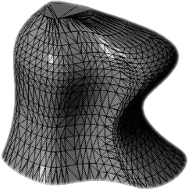###Stonelinks

Home | Posts | Projects | About

# Quickstart - Stoolbotics

When you fire up the simulator for the first time, you should see something like what is shown below.You’ll notice a robot is loaded into the simulator to start with. This simple three joint arm is called the Phantom Omni, and is defined by the `omni.json` file in the `robots` directory. All robot files that the simulator uses are described in such `robot.json` files in the `robots` directory. They are simple and easy to understand. Below we have reproduced `omni.json` as it is first loaded into the simulator:

``````"N" : "3",

"h1" : "z",
"h2" : "x",
"h3" : "x",

"q1" : ".1*t",
"q2" : ".1*cos(t)",
"q3" : ".001*t + .2*sin(t)",

"l1" : "40",
"l2" : "50",
"l3" : "50",

"P01" : "[0, 0, 0]",
"P12" : "[0, 0, l1]",
"P23" : "[0, l2, 0]",
"P3T" : "[0, l3, 0]",

"R01" : "rot(h1, q1)",
"R12" : "rot(h2, q2)",
"R23" : "rot(h3, q3)",
"R3T" : "eye(3, 3)"
``````

Let’s look at this file line by line to see how it makes a complete robot object:

• `N` is first declared to tell the simulator the number of joints to expect in this robot.
• All the joint axes are specified with an `h` and an index. In this case, shorthand is used (e.g. use of `z` instead of `[0, 0, 1]`), but if we wanted a non-standard axis vector we could have used something like `[-.1, .2, .4]`.
• Angle parameters are specified with a `q` and an index. These can be completely arbitrary functions of time, static numbers, or whatever you like. These parameters represent how much an axis has rotated or displaced along its axis.
• Link lengths are specified with an `l` and an index.
• Position vectors (`P` + index) tell the simulator how to get from one frame to the next. Prismatic joints can be created by including a joint axis parameter (a `q`) in the vector.
• Finally, rotation matrices (`R` + index) are specified by using the `rot()` command, which calculates the rotation matrix using the Euler-Rodriguez formula. If no rotation is desired, just specify the identity matrix with the `eye()` command. Sometimes, for static links it is necessary to specify extra frames that don’t have any rotation matrix. If this is the case, you would also just use the `eye()` command here.

All these variables can be changed once the simulator has started using the `set` command. There are many more commands available to you in the simulator that can be accessed through the command line. To see a list of them, just type `help` into the console and you should see a list like what’s below. To view help about a specific command, just type `help <command>`. A table showing what all the commands are, their syntax, and what they do is also included later on in this documentation.In the above case, we looked at the help for the `axis` command. Let’s see what it actually does:It is clear then that the axis command can be used to turn on and off the the axis for each intermediary joint coordinate frame. There are many other commands that can be used to manipulate the cosmetics of the simulation environment. These are all covered later in an example section on manipulating the environment. For now though, let’s check out another command, the `play` command. A simulator is pretty useless unless it can actually simulate things. The `play` command starts the simulation:To stop the simulator, just type `stop`. All the variables we set earlier are still modifiable during the runtime. To set these, we use the `set` command. For example, let’s set q3 to cos(t) by typing `set q3 cos(t)` into the command prompt:Though you can’t see it in a static picture, that joint is now moving pretty fast. Let’s use the `set` command again to slow it down. Type `set tscale .05` command and notice that it now goes slower. The value of an appropriate timescale may vary depending on how fast your computer is. `skew` mode can be enabled to rapidly adjust timescale as well as other parameters. For now though, just use `set`:This concludes the quickstart. To do more advanced things like play, record, manipulate the environment, drive the simulator from matlab, etc., check out the full documentation!